# CURRENT ARTICLES: VOLUME 22

## Generating Minimally Unsatisfiable Conjunctive Normal Forms »NBCDFPDF

Published October 29, 2020

A method of generating minimally unsatisfiable conjunctive normal forms is introduced. A conjunctive normal form (CNF) is minimally unsatisfiable if it is unsatisfiable and such that removing any one of its clauses results in a satisfiable CNF. Read More »

## Degree versus Dimension for Rational Parametric Curves »NBCDFPDF

Published September 22, 2020

Given a rationally parameterized curve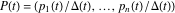in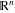or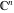, where the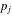and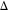are polynomials, we find the dimension of the smallest linear subset ofcontaining the curve. If all theandare of degreeor less, then it is known abstractly that this dimension isor less and rational normal curves play a key role in the argument. We consider this from a computational point of view with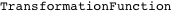playing an essential part in the discussion. Read More »

## Foundations of Computational Finance »NBCDFPDF

Published August 18, 2020

The Wolfram Language has numerous knowledge-based built-in functions to support financial computations. This article introduces many built-in and other financial functions that are based on concepts and models covered in undergraduate-level finance courses. Examples are taken from a wide range of finance areas. They emphasize importing and visualization of data from many sources, valuation, capital budgeting, analysis of stock returns, portfolio optimization and analysis of bonds and stock options. We hope that all the functions selected in this article are very useful for analyzing real-world financial data. All examples provide a unique set of tools for users to engage with real-world financial data and solve practical problems. The feature of automatic data retrieval from online sources and its analysis makes all results reproducible without any modifications in the code. We hope this feature will attract new users from the finance community. Read More »

## Sectional Curvature in Riemannian Manifolds »NBCDFPDF

Published March 19, 2020

The metric structure on a Riemannian or pseudo-Riemannian manifold is entirely determined by its metric tensor, which has a matrix representation in any given chart. Encoded in this metric is the sectional curvature, which is often of interest to mathematical physicists, differential geometers and geometric group theorists alike. In this article, we provide a function to compute the sectional curvature for a Riemannian manifold given its metric tensor. We also define a function to obtain the Ricci tensor, a closely related object. Read More »